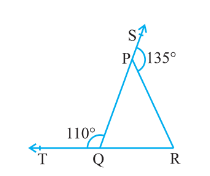Q

# In Fig. 6.39, sides QP and RQ of ∆ PQR are produced to points S and T respectively. If ∠ SPR = 135° and ∠ PQT = 110°, find ∠ PRQ.

1. In Fig. 6.39, sides QP and RQ of PQR are produced to points S and T respectively. If  SPR = 135° and PQT = 110°, find PRQ.ViewsGiven,
PQR is a triangle, SPR =,  PQT =

Now, TQP + PQR =  (Linear pair)
So, PQR =

Since the side of QP of the triangle, PQR is produced to S
So, PQR + PRQ =  (Exterior angle property of triangle)

Exams
Articles
Questions